[Next]: Probabilistic Cellular Automata
 [Up]: Project descriptions
 [Previous]: Gibbs measures of highly disordered systems
[Contents]   [Index]

## Interacting catalytic branching processes

Collaborator: K. Fleischmann , A. Sturm

Cooperation with: N.H. Barton (University of Edinburgh, UK), D.A. Dawson (Carleton University Ottawa, Canada), A.M. Etheridge (University of Oxford, UK), A. Klenke (Universität Köln), P. Mörters (University of Bath, UK), C. Mueller (University of Rochester, USA), L. Mytnik (Technion, Haifa, Israel), E.A. Perkins (University of British Columbia, Vancouver, Canada), J. Swart (Friedrich-Alexander-Universität Erlangen-Nürnberg), V.A. Vatutin (Steklov Mathematical Institute, Moscow, Russia), A. Wakolbinger (Johann Wolfgang Goethe-Universität Frankfurt), J. Xiong (University of Tennessee, Knoxville, USA)

Supported by: DFG Priority Program Interagierende Stochastische Systeme von hoher Komplexität'' (Interacting stochastic systems of high complexity)

Description: Pairs of interacting catalytic branching models describe the evolution of two materials which randomly move, split, and possibly disappear in space. The system is interactive in that the branching behavior of each material is dependent on the other one. This interaction destroys the usual independence assumption in branching theory, and requires new, different methods.

A major result this year is the construction of a competing species model with infinite variance . This is a breakthrough since up to date exclusively interacting branching models with finite variance branching have been constructed. In this case a competing species model has been treated in dimensions d<4 by Evans and Perkins , based on Barlow et al. . Besides others, their key tools had been a Girsanov-type theorem of Dawson , and a Tanaka-type formula for collision local times of some interacting critical continuous super-Brownian motions in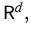d<6, from . But both tools are not available anymore if the finite variance assumption is dropped. In fact, Girsanov's theorem requires continuity in time of the martingale component; this is true only under finite variance. Moreover, Barlow et al.'s proof of the Tanaka formula relies on some uniform bound of continuous super-Brownian motion's mass in small balls (). This bound does not hold in our case, and validity of the Tanaka formula in the more general setup remains open. To prove convergence of approximating collision local times, we heavily apply a log-Laplace technique, originally used for collision local times in Mytnik . In our interacting model, an application of log-Laplace tools is still possible since we can represent the collision local time for the pair of interacting superprocesses with killing as a linear combination of collision local times for independent and conditionally independent measure-valued processes. This representation (which also holds for approximating collision local times) is based on the domination of interacting pairs by a pair of independent superprocesses. The domination property is an extension of an analogous result proved in  for finite variance super-Brownian motions.

A related line of research has been concerned with convergence of population systems to the heat equation with colored noise . In  rescaled branching particle systems in a random environment and associated lattice systems (infinite systems of stochastic differential equations) are shown to converge to solutions of the aforementioned stochastic partial differential equation (SPDE). Due to the correlation in the random environment, the resulting SPDEs are driven by a colored noise term. The latter can be investigated in higher dimensions. This is in contrast to white noise SPDEs which are restricted to dimension one. Apart from establishing an SPDE description of a class of particle systems, the particle picture itself and the approximation by systems of related SDEs provide a representation of a general class of SPDEs that also arise in other areas of application, for example in filtering theory. In our case, the approximation by a system of SDEs leads to a new existence result for the stochastic heat equation with colored noise and non-Lipschitz noise coefficients.

Motivated by investigations of Greven et al.  on linearly interacting Wright-Fisher diffusions on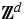catalyzed by a voter model, in  we study the extinction/explosion behavior in a supercritical super-Wright-Fisher diffusion on [0,1]. In , the long-term behavior in their two-dimensional model was expressed in terms of a binary splitting Wright-Fisher diffusion with rate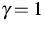(see Figure 1).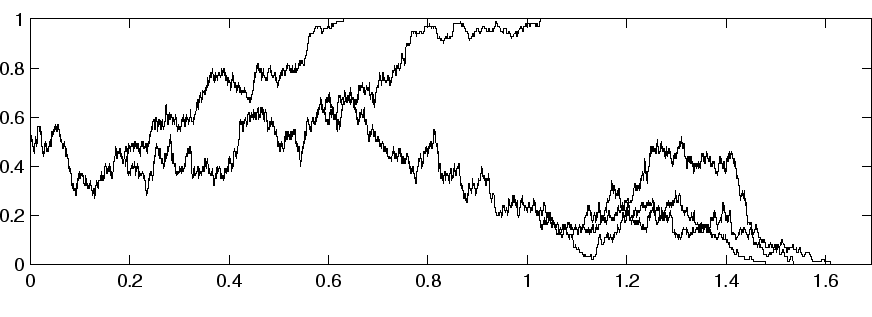We relate the latter model in a slightly more general case with the corresponding superprocess. Then the long-term behavior can be described completely: For rates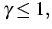mass in the interior (0,1) dies out after a finite random time, while for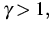the mass in (0,1) explodes with positive probability. In the case of explosion, the mass in (0,1) grows exponentially with rate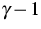and is approximately uniformly distributed over (0,1). These results are applied to show that the related semi-linear Cauchy problem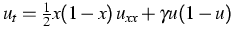on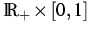has precisely four fixed points when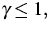and five when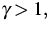and we determine their domain of attraction. In a supercritical branching particle system, the trimmed tree consists of those particles which have descendants at all times. We develop this concept in the superprocess setting. For a class of continuous superprocesses, we identify the trimmed tree, which turns out to be a binary splitting particle system with a new underlying motion that is a compensated h-transform of the old one. We show how trimmed trees may be estimated from above by embedded binary branching particle systems .

By studying a coalescent process in a random environment , progress has been made in  towards understanding one of the key issues in population genetics: the influence of selection, mutation and linkage (probable joint inheritance) on the genealogy of several genes. In this work, the distribution of the diffusion limit of the ancestry of a sample of fixed size is derived when the overall population size tends to infinity. The simplest case of such a diffusion limit--generally referred to as a coalescent because of its inverted tree structure--has been treated by Kingman . His coalescent and versions thereof have been used extensively in the analysis of population genetic data. Earlier work mostly considers a random sample from a population. This has made it difficult to model selection since the branching behavior of individuals depends on their genetic make-up, which hence has to be known. In order to incorporate general forms of selection we follow the genealogy of a sample conditioned on its genetic composition. For sample size two the limit is analyzed numerically. The analysis confirms the intuition that selection and linkage have a significant influence on the distribution of biologically relevant quantities that more simplistic models failed to capture.

A super-Brownian motion in two and three dimensions is constructed where particles'' give birth at a higher rate, if they approach the origin . This contradicts the intuition suggested by the fact that in more than one dimension Brownian particles do not hit a given point. Via a log-Laplace approach, the construction is based on Albeverio et al.  who calculated the fundamental solutions of the heat equation with one-point potential in dimensions less than four.

For a super-Brownian catalyst reactant pair in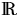, a difficult tightness problem has been resolved this year () leading to a universal mass-time-space functional scaling limit theorem on path space.

References:

1. S. ALBEVERIO, Z. BRZEZNIAK, L. DABROWSKI, Fundamental solution of the heat and Schrödinger equations with point interaction, J. Funct. Anal., 130 (1995), pp. 220-254.
2. M.T. BARLOW, S.N. EVANS, E.A.  PERKINS, Collision local times and measure-valued processes, Canad. J. Math., 43 (1991), pp. 897-938.
3. N.H. BARTON, A.M. ETHERIDGE, A.K. STURM, Coalescence in a random background, WIAS Preprint no. 756, 2002.
4. D.A. DAWSON, Geostochastic calculus, Canad. J. Statist., 6 (1978), pp. 143-168.
5. S.N. EVANS, E.A. PERKINS, Measure-valued branching diffusions with singular interactions, Canad. J. Math., 46 (1994), pp. 120-168.
6. K. FLEISCHMANN, A. KLENKE, J. XIONG, Pathwise convergence of a rescaled super-Brownian catalyst reactant process, Preprint, Universität zu Köln, Mathematisches Institut, 2002.
7. K. FLEISCHMANN, C. MUELLER, Super-Brownian motion with extra birth at one point, WIAS Preprint no. 790, 2002.
8. K. FLEISCHMANN, L. MYTNIK, Competing species superprocesses with infinite variance, WIAS Preprint no. 772, 2002.
9. K. FLEISCHMANN, J. SWART, Extinction versus explosion in a supercritical super-Wright-Fischer diffusion, WIAS Preprint no. 752, 2002, to appear in: Stochastic Process. Appl.
10.,Trimmed trees and embedded particle systems, WIAS Preprint no. 793, 2002.
11. A. GREVEN, A. KLENKE, A. WAKOLBINGER, Interacting Fisher-Wright diffusions in a catalytic medium, Probab. Theory Related Fields, 120 (2001), pp. 85-117.
12. J.F.C. KINGMAN, The coalescent, Stochastic Process. Appl., 13 (1982), pp. 235-248.
13. L. MYTNIK, Collision measure and collision local time for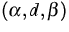superprocesses, J. Theoret. Probab., 11 (1989), pp. 733-763.
14. A.K. STURM, On convergence of population processes in random environments to the stochastic heat equation with coloured noise, WIAS Preprint no. 770, 2002, to appear in: Electr. J. Prob.

 [Next]: Probabilistic Cellular Automata
 [Up]: Project descriptions
 [Previous]: Gibbs measures of highly disordered systems
[Contents]   [Index]

LaTeX typesetting by I. Bremer
5/16/2003